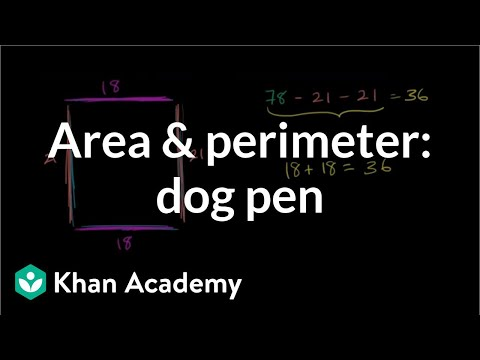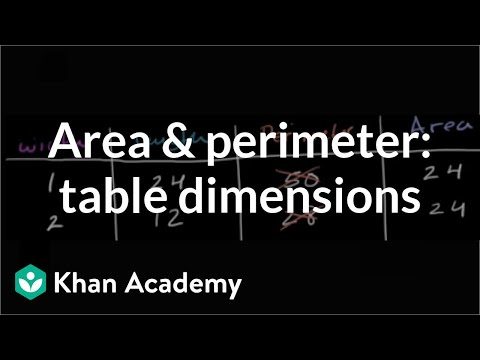Video

# Finding missing side when given area (Full video)

Description: Lindsay finds the missing side length on a rectangle when given the area of the rectangle. Well, maybe we don't know a special formula or a special equation to find width, but we do know one to find area of a rectangle, so let's use that formula and see how it can help us. We know that area of a rectangle can be found by multiplying the length of the rectangle, over here, the length, times the width. So if we multiply the two side lengths of a rectangle, we get its area.

### Other videos you might be interested in### Area & perimeter word problem: dog pen (Full video)